# Two theorems on square numbers

Nguyen Xuan Tho
Notes on Number Theory and Discrete Mathematics
Print ISSN 1310–5132, Online ISSN 2367–8275
Volume 28, 2022, Number 1, Pages 75–80
DOI: 10.7546/nntdm.2022.28.1.75-80

## Details

### Authors and affiliations

Nguyen Xuan ThoHanoi University of Science and Technology
Hanoi, Vietnam

### Abstract

We show that if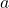is a positive integer such that for each positive integer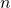,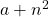can be expressed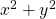, where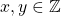, thenis a square number. A similar theorem also holds ifandare replaced by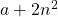and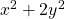, respectively.

### Keywords

• Elementary number theory
• Square numbers

• 11A15
• 11E04

### References

1. Ireland, K., & Rosen, M. (1998). A Classical Introduction to Number Theory (2nd ed.). Springer.
2. Wang, S. (1950). On Grunwald’s theorem, Annals of Mathematics, Second Series, 51(2), 471–484.